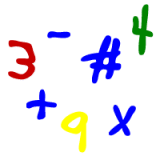# Kids Math

## Prime NumbersSkills needed:
Multiplication
Division
Whole numbers

What is a prime number?

A prime number is a whole number with exactly two factors, itself and 1.

Okay, maybe that's a bit hard to understand. Let's take a look at some examples:

The number 5 is a prime number because it cannot be divided evenly by any other numbers except for 5 and 1.

The number 4 is not a prime number because it can be divided evenly by 4, 2, and 1.

Is the number 13 a prime number?

It can't be divided by 2, 3, 4, 5, 6, 7, 8....etc. Only by 1 and 13. Yes, 13 is a prime number.

Is the number 25 a prime number?

It can't be divided by 2, 3, 4....true. Ah, but it can be divided by 5, so it is not a prime number.

Here is a list of the prime numbers between 1 and 100:

2, 3, 5, 7, 11, 13, 17, 19, 23, 29, 31, 37, 41, 43, 47, 53, 59, 61, 67, 71, 73, 79, 83, 89, 97

Take a look at a few of them and see if you can figure out any other number that they can be divided by other than the number itself or the number 1. (hint: we promise the answer is "no" and they are, therefore, prime numbers).

Some tricks to prime numbers:
• The number 1 is not considered a prime number.
• All even numbers greater than 2 are not prime numbers.
• There are an infinite number of prime numbers.
• Prime numbers are often used in cryptography or security for technology and the internet.
• The number 1 used to be considered a prime number, but it generally isn't anymore.
• The largest prime number known has around 13 million digits!
• The Greek mathematician Euclid studied prime numbers in 300BC.
• The number 379009 is a prime number. It also looks like the word Google if you type it into a calculator and look at it upside down!
• Here is a interesting sequence of prime numbers in which all of the digits have circles in them:
• 6089
• 60899
• 608999
• 6089999
• 60899999
• 608999999

The fundamental theorem of arithmetic says that any number can be expressed by a unique product of prime numbers.

Back to Kids Math

Back to Kids Study